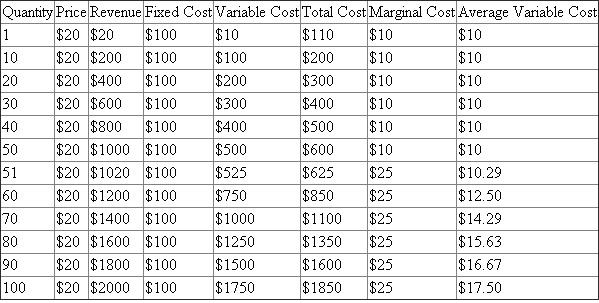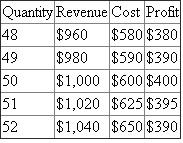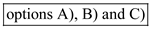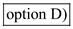# Quiz 8: Behavioral Economics

Business

Putting into a table the information that we are given for selected values, we have:Note that quantity numbers that are chosen for the above table is arbitrary. The key thing is to be able to see how things change when the quantity changes from 50 to 51. For a competitive firm, price is always determined by the market equilibrium, which in this problem is \$20. The revenue can be calculated by multiplying the price of each units sold (\$20) by the number of units sold (Quantity). Fixed cost is constant at \$100 because by definition, fixed cost does not change with the quantity. Variable cost is calculated as given in the problem, \$10 per unit until the 50 th unit, at which point it becomes \$25 per unit after. Total cost is simply the sum of the fixed cost and variable cost. Marginal cost is the change in the total cost, which is always going to be \$10 for the first 50 units (because the variable cost is the only thing that changes in total cost, and it increases by \$10 for every new unit produced) and for units after the 50 th , it will be \$25. Average variable cost is calculated by taking variable cost and dividing by the quantity. First, we see that price, which is \$20, does not exceed the average variable cost for the first 50 units, nor will it exceed the price when quantity iS100. After the 50 th unit, we see that average variable cost is increasing, so eventually, the average variable cost will exceed price. However, this will happen after 100 units are produced. The marginal cost per unit for the first 50 units, as we calculated, is \$10. This is because the only thing that is changing in total cost is variable cost, which means that the change in total cost is the same as the change in variable cost, which is \$10 for the first 50 units. Similarly, for units 51 and higher, the variable cost will be \$25, so now each additional unit produced will add \$25 to the total cost, which means marginal cost is \$25. Profit is maximized when marginal cost is equal to marginal revenue. For a perfectly competitive firm, the price is always equal to the marginal revenue, which means that marginal revenue is always \$20. Marginal cost is \$10 for units 50 and below, and \$25 for units 50 and above, so we know that marginal cost must be \$20 at some point between a quantity of 50 and 51. Calculating the profit around Q=50, we have:We see that profit is increasing until Q=50, at which point it starts decreasing. So, the firm will get the largest profit if it sets its output level at Q=50.

Economists classify industries into four basic models based on their market structures: pure competition, pure monopoly, monopolistic competition, and oligopoly. Purely competitive industries are characterized by a large number of firms producing a standardized product. No firm is able to influence the market price. Monopoly: One firm as the sole seller of a product characterizes pure monopoly industries. There will be no close substitutes for the product. Entry into the industry is blocked. It is a price maker. Monopolistically competitive industries are characterized by a relatively large number of firms producing differentiated products. These firms compete largely through nonprice competition by differentiating their products with advertising, design, and workmanship. Oligopoly involves only a few sellers of a product, and each firm is affected by the decisions of the others, and takes this into account when setting price and output. A supermarket in my hometown: Oligopoly Super markets are few in number, and each firm supplies has its set of market share, and pricing decisions are done based on the prices set by other supermarkets. The steel industry: Oligopoly, Steel industry can be characterized as an oligopoly, because a small number of large firms dominate the market and must take into account one another's business decisions. In recent years, competition in the steel industry has increased. A wheat farm: Perfect Competition, Is part of a purely competitive industry. There are a very large number of wheat producers, each with little or no market power, and they all produce a standardized product. The commercial bank: Monopolistic There will be many banks, and they differentiate their product through branding and variation in service offering. Ex: Processing fee for a loan is varied across the banking industry. A bank may wave off the processing fee for some segments of its customers. But others may charge. The automobile industry is clearly characterized by oligopoly. There are a relatively few number of firms. Firms differentiate their products with advertising, design, and workmanship. Market entry is difficult as these suppliers have a substantial market share. Recent opening up of US economy for auto imports has made this sector more competitive.

Firms in perfect competition produce identical goods such that consumers are in different from buying from different sellers. Due to this reason, no firm is able to influence the market price which is therefore, determined by the overall market demand and supply function. In this manner, all firms are considered as price takers. When each of the firm in the market increases production, or there are new firms entering the market, the total supply is increased. This shift the supply curve to the right reducing the price. Thus, it is true that price should fall when the output is increased, but this does not provide explanation for the horizontal marginal revenue curve. Law of diminishing marginal utility indicates that marginal utility continues to decline, and thus, is downward sloping. Here also, diminishing marginal utility does not explain the shape of the marginal revenue curve. For competitive firm, the demand faced by individual firm is perfectly elastic, but the market demand function is downward sloping. Hence, optionsare incorrect. Note that marginal revenue is the additional revenue received for each additional unit sold. Competitive firm charges the same price for the product sold by it because they are price takers. They receives the same marginal revenue for any quantity sold. Therefore, the marginal revenue curve is horizontal showing a constant marginal revenue. Hence,is correct.